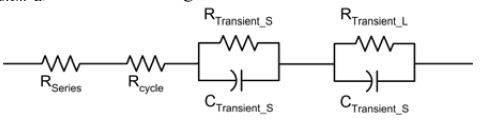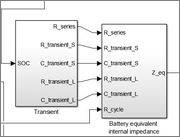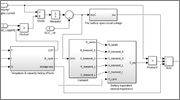# The battery equivalent internal impedance equation

marrcko

## Homework Statement

Hello, long story short: I need to create a model of Li-Ion battery and I'm stuck on calculating the battery equivalent internal impedance. The thing is, I don't know the equation or even how to make one. However, I have a circuit drawing:## Homework Equations

Values in circuit are calculated by other equations, and I don't think they are relevant here.

## The Attempt at a Solution

I have nothing to start of except the circuit drawing, so naturaly I don't have any attempts. Well, I tried to draw the circuit with simulink, but values for those blocks are not dinamical.
Could someone explain how to calculate or write down an equation for battery equivalent internal impedance
Edit: After googling and trying to remember what i learned from school, I think it should be r =Rseries + Rcycle +1/R_transient_s + 1/R_transient_L

Am I right? But Does capacitors don't have any influence for r?

Last edited:

For what course is this problem. It is curious that you are given a problem for which you are not prepared to solve.

marrcko
For what course is this problem. It is curious that you are given a problem for which you are not prepared to solve.
For Mathematical modeling of engineering systems course :).

Its for individual assignment. We had to find an article with some matlab/simulink model and try to simulate something with it.

All other equations are given in the article, except this.

Edit: I did not want to double post
I assume that internal impedance = Internal resistance?

Battery is DC so Internal resistance of it should be R=R1+R2+... if I remember correctly. So, i have all R values, so maybe I just should sum them? Don't know what to do with capacitors thoughLast edited:
What specifically do you want to determine?

Gold Member
For Mathematical modeling of engineering systems course :).

Its for individual assignment. We had to find an article with some matlab/simulink model and try to simulate something with it.

All other equations are given in the article, except this.

Edit: I did not want to double post
I assume that internal impedance = Internal resistance?

Battery is DC so Internal resistance of it should be R=R1+R2+... if I remember correctly. So, i have all R values, so maybe I just should sum them? Don't know what to do with capacitors thoughresistance is the DC impedance like you said.

But when the load changes, the voltage source will no longer be DC.
Lets take a Li-on battery powering an inverter that drives a motor. There will be all sorts of nasty harmonics on the voltage line that is SUPPOSED to be DC.

The impedance is going to change based on frequency. Finding the equivalent resistance is easy, if you know how to do laplace and work in the frequency domain.
What kind of background do you have? What class is this for.

If you are modeling the entire LI-on battery, including SOC and Voc, etc... I recommend you use state space modeling. It can provide a simple way to manipulate and present the data.

•marrcko
marrcko
What specifically do you want to determine?
In this picture, there are 2 subsystems in this model (simulink). For first one, I have equations to calculated all R and C values. However, in second subsystem I need to calculate a Z_eq in given values. Here Z_eq is battery equivalent internal impedance [ohm].If you are modeling the entire LI-on battery, including SOC and Voc, etc... I recommend you use state space modeling. It can provide a simple way to manipulate and present the data.
The catch for this task is that I have to use same model that is used in the article (see picture below). And the only issue here is subsystem with missing equation. Using state space require atlest 2 matrices (other 2 is eye(n) and zeros(n,m)). And this model uses only scalar values e.g. C_transient_S (SOC) = 752.9 x exp(-13.51 x SOC) + 703.6On the other hand, I should consider you suggestion as Plan B, cause it looks possible to adapt the model to use state space instead of that subsystem (adding mux and etc..)

Last edited:
Gold Member
In this picture, there are 2 subsystems in this model (simulink). For first one, I have equations to calculated all R and C values. However, in second subsystem I need to calculate a Z_eq in given values. Here Z_eq is battery equivalent internal impedance [ohm].The catch for this task is that I have to use same model that is used in the article (see picture below). And the only issue here is subsystem with missing equation. Using state space require atlest 2 matrices (other 2 is eye(n) and zeros(n,m)). And this model uses only scalar values e.g. C_transient_S (SOC) = 752.9 x exp(-13.51 x SOC) + 703.6Given the information that you have I would assume DC resistance.
Although this will not be as accurate as second or third order model, it is as close as you can get following the block diagram you are given.

marrcko
Given the information that you have I would assume DC resistance.
Although this will not be as accurate as second or third order model, it is as close as you can get following the block diagram you are given.
If I may, I would like to (not sure yet :-) ) add your note to presentation.
So Z_eq =Rseries + Rcycle +1/R_transient_s + 1/R_transient_L then?

Gold Member
Z_eq =Rseries + Rcycle +1/R_transient_s + 1/R_transient_L

what are the units of Z_eq?

what are the units of the equation you gave?

marrcko
what are the units of Z_eq?

what are the units of the equation you gave?
Right.. the units.. now it makes no sense. Z_eq unit is Ohm. And in this "equation" it will be [Ohm] +[Ohm] + 1/[Ohm] +1/[Ohm]

So Z_eq =Rseries + Rcycle +1/R_transient_s + 1/R_transient_L then?

this would be incorrect. the units of z are ohms. your equation is adding ohms to reciprocal ohms.

•donpacino
marrcko
this would be incorrect. the units of z are ohms. your equation is adding ohms to reciprocal ohms.
Did some googling again and found out that R (in this case z) should be calculated:
1/Rrc = 1/R_transient_s + 1/R_transient_L, where Rrc is tmp variable
Z_eq = Rseries + Rcycle +Rrc

Gold Member
Did some googling again and found out that R (in this case z) should be calculated:
1/Rrc = 1/R_transient_s + 1/R_transient_L, where Rrc is tmp variable
Z_eq = Rseries + Rcycle +Rrc
1/Rrc = 1/R_transient_s + 1/R_transient_L
This would be true if the resistors were in parallel with each other.

marrcko

This would be true if the resistors were in parallel with each other.
My what? You mean how much do I know about electrical "stuff"? not much.

Edit. Yeah... No I see my problem. the capacitors are confusing me. So technically, resistors are in series, aren't?

I know nothing of Simulink or its application and use. For what it is worth If I did the math correctly I think the Equivalent resistance of the six component = Rs + Rcycle + RTS(1-e-t/CTSRTS) + RTL (1-e-t/CTLRTL) as a function of time from the moment current begins to flow.

But for time t >> RTSC and RTL the equivalent resistance is just Rseries + Rcycle + RTS + RTL

Note that R⋅C where R is in ohms and C is in farads has units of time in seconds.

Does any of this look familiar?

•marrcko
marrcko
My what? You mean how much do I know about electrical "stuff"? not much.
I know nothing of Simulink or its application and use. For what it is worth If I did the math correctly I think the Equivalent resistance of the six component = Rs + Rcycle + RTS(1-e-t/CTSRTS) + RTL (1-e-t/CTLRTL) as a function of time from the moment current begins to flow.

But for time t >> RTSC and RTL the equivalent resistance is just Rseries + Rcycle + RTS + RTL

Note that R⋅C where R is in ohms and C is in farads has units of time in seconds.

Does any of this look familiar?
Yes, this look familiar and makes new thoughts about the solution. I will report back if this succeeded during this week. Thank you

Gold Member
Yes, this look familiar and makes new thoughts about the solution. I will report back if this succeeded during this week. Thank you
So in simulink, the way you have the system setup, you cannot have a dependence on time for equivalent impedance. Just an FYI

My what? You mean how much do I know about electrical "stuff"? not much.

Edit. Yeah... No I see my problem. the capacitors are confusing me. So technically, resistors are in series, aren't?
Yes you are correct!